Dedicated to those who never had the opportunity to receive a good education
Dedicated to those who never had the opportunity to receive a good education
0%

# Energy threshold for creation of particles in relativistic collisions

In the present article, we consider the collision of two particles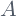, and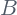, withat rest in the lab frame of reference before the collision. As a result of the collision one or more particles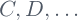, are produced. The produced particles can be, in principle, different from the colliding ones. Such kind of reaction will take place when, before the collision, there is enough energy. This means that there must be a minimal quantity of energy available, in order for the reaction to take place. This minimal energy is called the energy threshold of the reaction.  We will find the threshold for such kind of reactions. For this end, we first need to recall the invariant associated with energy and momentum.

## Lorentz invariant energy-momentum relation

Let’s start by recalling the connection between the energy, momentum and rest energy of a particle whose rest mass is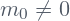. The total energy of the particle is

(1)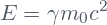The momentum is

(2)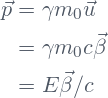We can see in Eq.(2) that for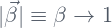we get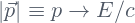. Also note that, according to Eq.(1) we find that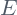increases to very large values when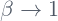. This is so because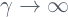for. We conclude that at very large energies, particles whose rest mass is not zero, will have a momentum-energy relation very close to that for the massless particles.

With help of the expression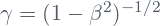, we can rewrite Eq.(1) in the form

(3)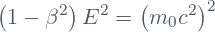Now we can use the last equality in Eq.(2), i.e.,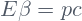, in order to rewrite Eq.(3) as follows:

(4)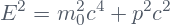The latter equation seems to be simply a mere combination of Eqs(1) and (2). However, an additional important fact is made evident if we rewrite Eq.(4) in the form

(5)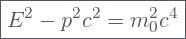This last form says that the combination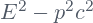, gives the same value in all inertial reference systems, since the quantity on the right side, i.e.,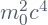, is invariant under Lorentz transformations. This invariant connection between energy and momentum is very useful in the analysis of relativistic collisions.

## Reaction threshold

Let’s consider the case of a particlewhose rest mass is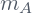, which collides with the particle, whose rest mass is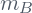, and which is at rest in the lab frame. As a result of this collision, several particles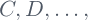could be produced.

(6)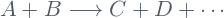The rest mass of each one of the products is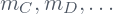. The question to be answered is: Assuming that the reaction is allowed from the point of view of conservation laws being satisfied, what is the minimal energy of the particlefor the reaction to be achieved? This minimal energy for the particleis called the energy threshold of the reaction.The particle is at rest in the lab frame. The energy threshold of the incident particle , for the reaction to be achieved, depends on the rest mass of the produced particles

In answering questions regarding minimal conditions of energy, it is necessary to work in the center of mass frame of the colliding particles. Why? because by definition, in the center of mass frame, the center of mass itself remains at rest. This means that the contribution of the produced particles to the total energy comes from their rest masses together with their velocities with respect to the center of mass. Therefore, the minimal condition is achieved when all of the produced particles are at rest in the center of mass frame.

However, the Energy we are looking for has to be calculated, not in the center of mass frame, but in the lab frame. This means that we need to work with these two reference frames simultaneously. This would be awkward if there were not for the energy-momentum relation given in Eq.(5), as will we see.

Let’s start by calculating the total energy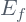and momentum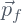, of the produced particles in the center of mass frame. Clearly, if all of them are at rest, then the individual, as well as the final total momentum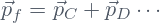, are zero, while the final total energy is simply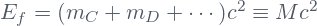. Therefore, the energy-momentum relation Eq.(5) reduces to:

(7)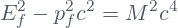Now let’s calculate the total energy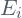and momentum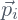, before the collision occurs. This calculation will be made in the lab frame. The initial total energy is the sum of the total energy of both particles, namely,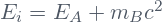. Remember that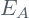is what we are trying to calculate. The initial total momentum is the sum of the momentum of the colliding particles. But, the particleis at rest. Therefore, the initial total momentum is just the momentum of the particle, i.e.,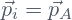. Now we can calculate the energy-momentum relation Eq.(5):

(8)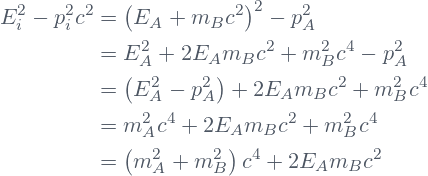Now comes the marvelous thing. We have calculated the energy-momentum relations after and before the collision. Those calculations were performed in two different reference systems, namely, the center of mass frame, and the lab frame, respectively. But those quantities have to be the same in all inertial reference systems! Therefore we just have to equal them to solve our problem! Therefore, the answer we are looking for follows from equaling Eqs.(7) and (8):

(9)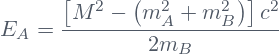Note that if after the collision, the only particles are the sameand, then we will have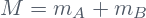, and therefore, Eq.(9) will reduce to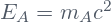, i.e., the incident particlehas to be at rest, asis before the collision. This is logically clear. the particles have just ‘to exist’ before the ‘collision’, in order to be ‘produced’ after the collision.

Share it!